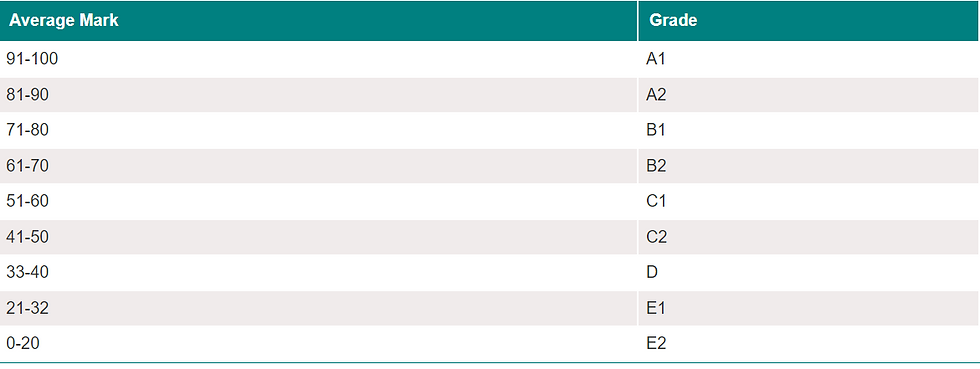top of page

# Calculate Grade of students in Final Exams

In this blog, we are going to talk about How to calculate the overall grade of the student in the final exams.Our python application depends on taking input from the user in the 6 subjects by using the following line code

```print("Enter Your Marks in 6 Subjects: ")
markOne = float(input())
markTwo = float(input())
markThree = float(input())
markFour = float(input())
markFive = float(input())
markSix = float(input())```

The output will be like this:

```Enter Your Marks in 6 Subjects:
50
57.5
38
91
85
70```

We take these inputs and we calculate the average by the following line code:

```total =markOne+markTwo+markThree+markFour+markFive+markSix
avg = total/6```

After that, we pass the avg to the cal_grade function to calculate the grade of the student

`cal_grade(avg)`

The cal_grade function calculates the grades as shown in the following code

```def cal_grade(input_avg):
if input_avg>=91 and input_avg<=100:
elif input_avg>=81 and input_avg<91:
elif input_avg>=71 and input_avg<81:
elif input_avg>=61 and input_avg<71:
elif input_avg>=51 and input_avg<61:
elif input_avg>=41 and input_avg<51:
elif input_avg>=33 and input_avg<41:
elif input_avg>=21 and input_avg<33:
elif input_avg>=0 and input_avg<21:
else:
print("Invalid Input!")```

The output for the previous inputs will be

`Your Grade is B2`

you can refer to the complete code from the following link